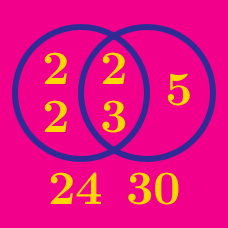Number Theory

# Greatest Common Divisor

What is the common divisor of $$33$$ and $$55$$ except $$1$$?

Simplify$\dfrac{2^2 \times 3}{2 \times 3 \times 5} .$

What is the greatest common divisor of 35 and 91?

What is the gcd(A, B) if:

A = $$6 \times 35,$$ and
B = $$8 \times 15?$$

Simplify $\dfrac{15}{20}.$

Details and assumptions:
- You may use the fact that $$15= 3 \times 5$$ and $$20 = 4 \times 5$$.

×